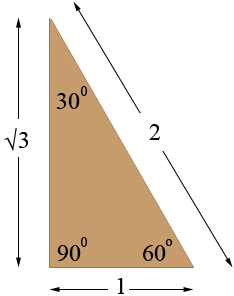SEARCH HOMEMath Central Quandaries & QueriesQuestion from Ron: 30-60-90 triangle. Base is 2.75 how do I find the length of the other two sides? Thanks in advance.Hi Ron,

It depends on which side is the base. If the side opposite the 30 degree angle in a 30-60-90 triangle has length 1 unit then the hypotenuse has length 2 units and the third side has length √3 units.Since any other 3-60-90 triangle is similar to this one the ratio of the side measurements remains the same. Thus if a 30-60-90 triangle has the side opposite the 30 degree angle of length 17.2 metres then the hypotenuse has length 2 × 17.2 = 34.4 metres and the third side of length √3 × 17.2 = 29.8 metres.

Likewise if a 30-60-90 triangle has the side opposite the 60 degree angle of length 17.2 metres then the side opposite the 60 degree angle has length 17.2/√3 = 9.9 metres and the hypotenuse has length 2 × 9.93 = 19.9 metres.

I hope this helps,
PennyMath Central is supported by the University of Regina and The Pacific Institute for the Mathematical Sciences.